Next: Sample session with cdd+ Up: Voronoi Diagram and Delaunay Previous: What is the Delaunay   Contents

## Computing the Delaunay complex and the Voronoi diagram. What does it mean and how to do it with available software?

Let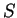be a given set of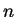points in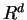. Computing the Voronoi diagram normally means to generate the set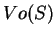of Voronoi vertices, and computing the Delaunay complex is essentially the same thing. Once the Voronoi vertices are generated, the nearest neighbor sets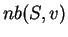for all Voronoi vertices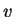can be easily computed, and in fact most of the algorithms for generating the Voronoi vertices computes the nearest neighbor sets as well at the same time.

The complexity of computing the Voronoi diagram is not well understood in general. For example, there is no known algorithm that runs polynomial in the size of input and output. For the much easier nondegenerate case, there is an algorithm, known as the reverse search algorithm, which runs in time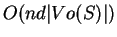. When the dimension is fixed (in particular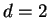), one can analyse complexities of various-type algorithms in terms of the input size. In the plane, there are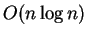algorithms that is optimal, and for fixed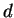there is an incremental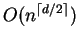algorithm, see [Ge97, Chapter 20].

How large is the number |Vo(S)| of output? The tight upper bound was given in [Sei91] which is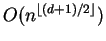. While this bound may be a far over-estimate of expected behavior, the number of output typically grows exponentially inand, and thus the computation itself is expected to be heavy. Therefore, one must take a caution to do the Delaunay/Voronoi computation. In fact,

I know quite a few people who tried to use Voronoi diagram computation codes in order to accomplish a much simpler task.
It is not only a waste of time and computer resources, but it often leads to a prohibitively hard computation, while an appropriate use of mathematical techniques resolves the problem instantly.

For example, the following computations are much simpler and should be solved via linear programming techniques in Section 4:

• For given two points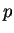and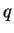in, check whether their Voronoi cells are adjacent in the Voronoi diagram, see 3.5.
• For any given point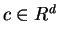, find a Delaunay cell containing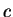, see 3.7.

The most natural way to compute the Voronoi diagram is by computing the vertices and the extreme rays of the polyhedron in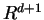given in 3.2. By ignoring the last component of each vertices we obtain the Voronoi vertices.

SubsectionsNext: Sample session with cdd+ Up: Voronoi Diagram and Delaunay Previous: What is the Delaunay   Contents
Komei Fukuda 2004-08-26